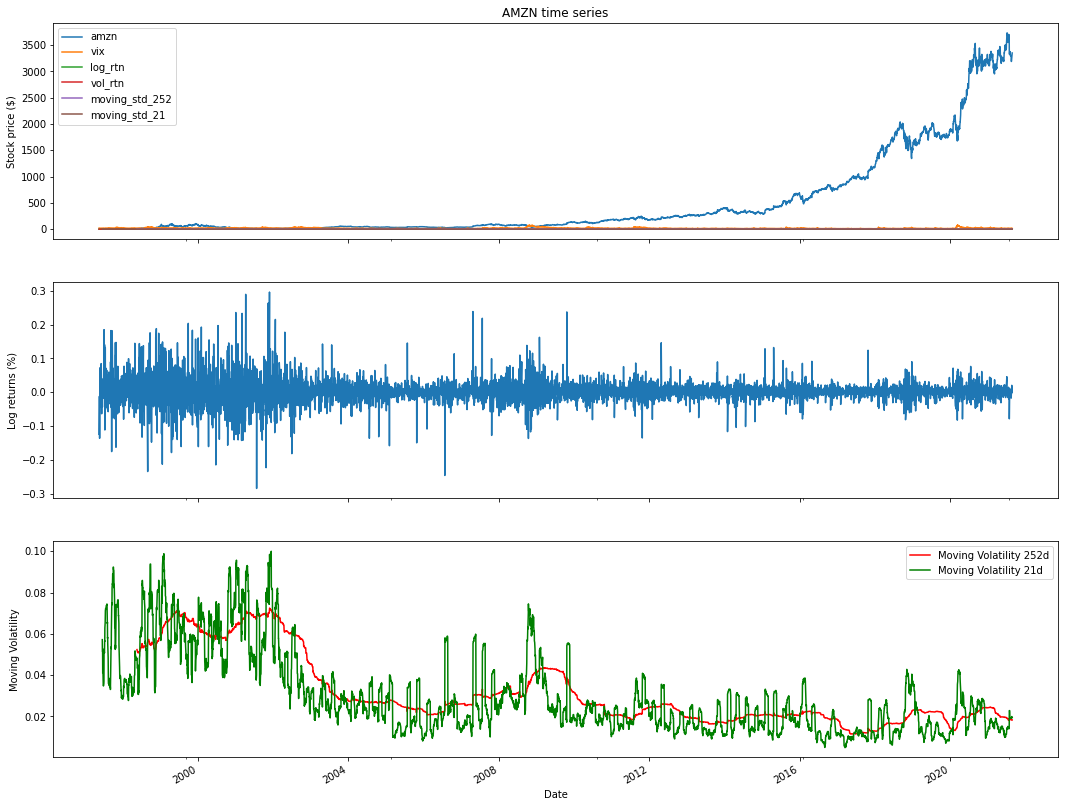# Amazon stock price analysis in python

## Amazon(AMZN) focuses on e-commerce, cloud computing, etc

Analyzing Amazon's stock price using Python involves collecting historical price data, performing data analysis, and creating visualizations to gain insights into the company's stock performance. Here's a step-by-step guide on how to conduct Amazon stock price analysis in Python:

Import Libraries:
Start by importing the necessary Python libraries for data manipulation, analysis, and visualization. Commonly used libraries include pandas, numpy, matplotlib, and yfinance to fetch historical data:

Python
Copy code
import pandas as pd
import numpy as np
import matplotlib.pyplot as plt
import yfinance as yf
Data Retrieval:
Use the yfinance library or other financial data sources to fetch historical data for Amazon's stock. Specify the start and end dates for the data you want to analyze:

Python
Copy code
Data Exploration:
Explore the fetched data to understand its structure and contents. Use functions like head(), tail(), describe(), and info() to inspect the dataset:

Python
Copy code
Data Visualization:
Create visualizations to analyze the historical performance of Amazon's stock. Common visualizations include line charts to visualize price movements:

Python
Copy code
plt.figure(figsize=(12, 6))
plt.title('Amazon Stock Price')
plt.xlabel('Date')
plt.ylabel('Price')
plt.legend()
plt.show()
Technical Analysis (Optional):
Perform technical analysis by calculating and visualizing technical indicators like moving averages, relative strength index (RSI), and MACD. Libraries like ta-lib can be used for these calculations.

Statistical Analysis (Optional):
Conduct statistical analysis to calculate summary statistics, volatility measures, and correlations with other assets. numpy and pandas are useful for these calculations.

Sentiment Analysis (Optional):
Consider incorporating sentiment analysis of news articles or social media data related to Amazon to understand market sentiment's impact on the stock price.

Fundamental Analysis (Optional):
Analyze fundamental factors affecting Amazon, such as earnings reports, revenue growth, and market share, which can influence the stock's performance.

Prediction and Forecasting (Optional):
You can use time series forecasting techniques like ARIMA or machine learning models to make predictions about future Amazon stock price movements.

Risk Management and Decision Making:
Based on your analysis, formulate investment strategies, set risk management parameters, and make informed investment decisions regarding Amazon's stock.

Keep your analysis up to date with the latest data to adapt to changing market conditions and make timely decisions.

Remember that investing in stocks carries risks, and it's crucial to do thorough research, consider factors like company news and market trends, and potentially consult with financial experts before making investment decisions based on your analysis of Amazon's stock price or any other stock.

What are alternative sources to get financial data?

There are a number of alternative sources like Quandal, intrinsic, google others.

!pip install yfinance
import pandas as pd
import numpy as np
import yfinance as yf

### How to get  AMZN financial data from Yahoo Finance in Python?

start='2020-01-01',
end='2021-08-28',
progress=False)
df.tail(9)
import matplotlib.pyplot as plt

### How to plot Amazon's close price?

df['Close'].plot(figsize=(12,8))
<matplotlib.axes._subplots.AxesSubplot at 0x7f2c61f1cc50>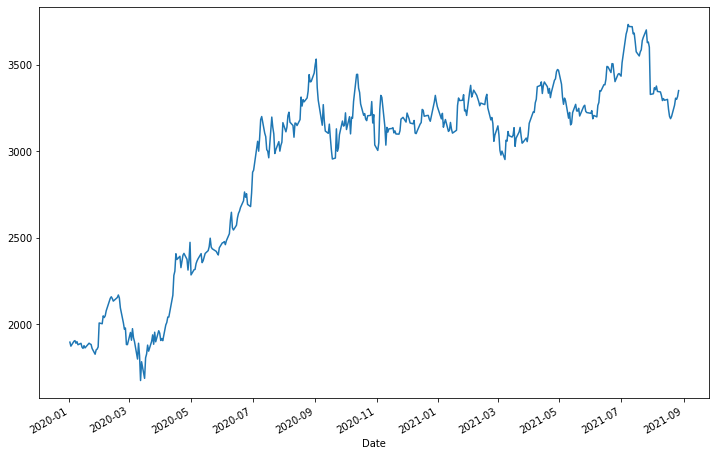## How to plot Amazon close price volume?

df[['Close''Volume']].plot(subplots=True, style='b',
figsize=(128))
array([<matplotlib.axes._subplots.AxesSubplot object at 0x7f2c61e14c90>,
<matplotlib.axes._subplots.AxesSubplot object at 0x7f2c61e39590>],
dtype=object)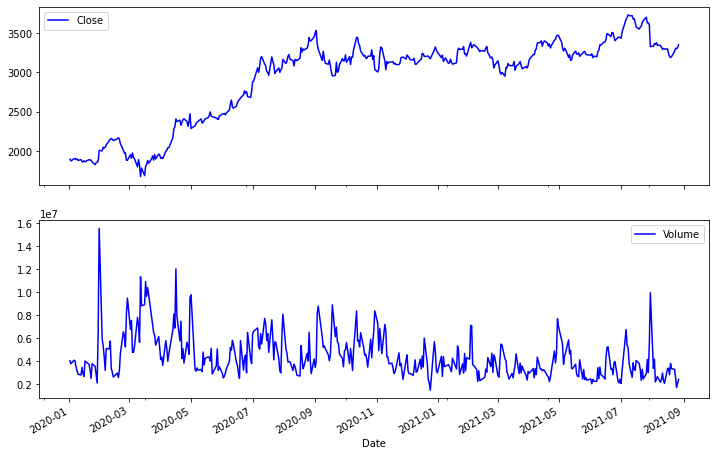### How to describe Amazon mean median and other statistics?

df.describe()

df['simple_rtn'] = df.Close.pct_change()
df['log_rtn'] = np.log(df.Close/df.Close.shift(1))
df['log_rtn'].plot(subplots=True, style='b',
figsize=(128))
array([<matplotlib.axes._subplots.AxesSubplot object at 0x7f2c61899a10>],
dtype=object)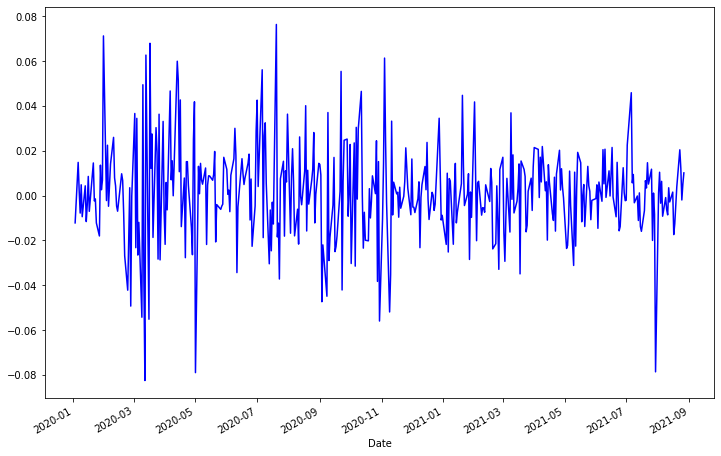### How to convert AMZN Stock prices into log and simple returns in Python?

df['log_rtn'].tail(12)
Date 2021-08-12 0.003454 2021-08-13 -0.002889 2021-08-16 0.001523 2021-08-17 -0.017438 2021-08-18 -0.012646 2021-08-19 -0.004217 2021-08-20 0.003820 2021-08-23 0.020391 2021-08-24 0.012146 2021-08-25 -0.001999 2021-08-26 0.005085 2021-08-27 0.010091 Name: log_rtn, dtype: float64
from plotly.offline import iplot, init_notebook_mode
init_notebook_mode()

## How to plot log returns in Python?

df_rolling = df[['simple_rtn']].rolling(window=21) \
.agg(['mean''std'])
df_rolling.columns = df_rolling.columns.droplevel()
df_outliers = df.join(df_rolling)
import pandas as pd
import numpy as np
import yfinance as yf
import seaborn as sns
import scipy.stats as scs
import statsmodels.api as sm
import statsmodels.tsa.api as smt

### How to compare stock price and vix?

start='1985-01-01',
end='2021-08-28',
progress=False)
df.columns = df.columns.droplevel(0)
df = df.rename(columns={'AMZN''amzn''^VIX''vix'})
df.tail()
df['log_rtn'] = np.log(df.amzn / df.amzn.shift(1))
df['vol_rtn'] = np.log(df.vix / df.vix.shift(1))
df.dropna(how='any', axis=0, inplace=True)
corr_coeff = df.log_rtn.corr(df.vol_rtn)
corr_coeff = df.log_rtn.corr(df.vol_rtn)
ax = sns.regplot(x='log_rtn', y='vol_rtn', data=df,
line_kws={'color''red'})
ax.set(title=f'AMZN vs. VIX ($\\rho$ = {corr_coeff:.2f})',
ylabel='VIX log returns',
xlabel='AMZN log returns')
[Text(0, 0.5, 'VIX log returns'),
Text(0.5, 0, 'AMZN log returns'),
Text(0.5, 1.0, 'AMZN vs. VIX ($\\rho$ = -0.33)')]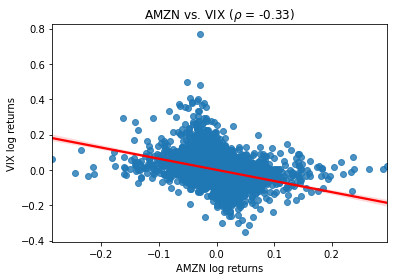### How to plot distribution of stock price and Q-Q plot?

r_range = np.linspace(min(df.log_rtn), max(df.log_rtn), num=1000)
mu = df.log_rtn.mean()
sigma = df.log_rtn.std()
norm_pdf = scs.norm.pdf(r_range, loc=mu, scale=sigma)
fig, ax = plt.subplots(12, figsize=(168))
# histogram
sns.distplot(df.log_rtn, kde=False, norm_hist=True, ax=ax)
ax.set_title('Distribution of AMZN returns', fontsize=16)
ax.plot(r_range, norm_pdf, 'g', lw=2,
label=f'N({mu:.2f}{sigma**2:.4f})')
ax.legend(loc='upper left');
# Q-Q plot
qq = sm.qqplot(df.log_rtn.values, line='s', ax=ax)
ax.set_title('Q-Q plot', fontsize = 16)
/usr/local/lib/python3.7/dist-packages/seaborn/distributions.py:2557: FutureWarning:

distplot is a deprecated function and will be removed in a future version. Please adapt your code to use either displot (a figure-level function with similar flexibility) or histplot (an axes-level function for histograms).


Text(0.5, 1.0, 'Q-Q plot')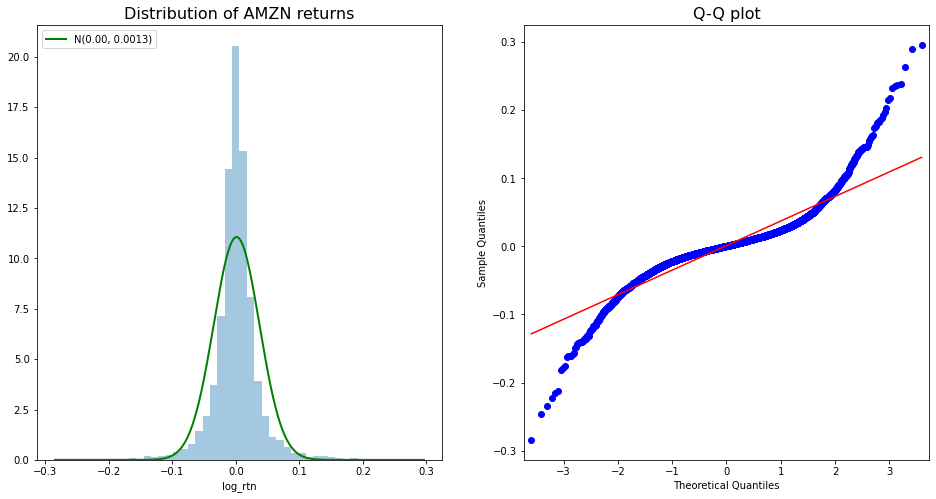df.log_rtn.plot(title='Daily AMZN returns')

### How to plot daily Amazon stock price?

<matplotlib.axes._subplots.AxesSubplot at 0x7f2c3ecc2e10>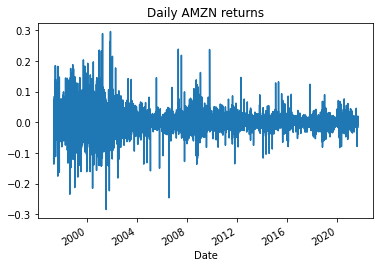### How to plot absolute return and autocorrelation?

N_LAGS = 50
SIGNIFICANCE_LEVEL = 0.05
acf = smt.graphics.plot_acf(df.log_rtn,
lags=N_LAGS,
alpha=SIGNIFICANCE_LEVEL
)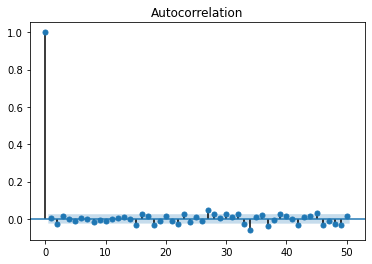fig, ax = plt.subplots(21, figsize=(1210))
smt.graphics.plot_acf(df.log_rtn ** 2, lags=N_LAGS,
alpha=SIGNIFICANCE_LEVEL, ax = ax)
ax.set(title='Autocorrelation Plots',
ylabel='Squared Returns')
smt.graphics.plot_acf(np.abs(df.log_rtn), lags=N_LAGS,
alpha=SIGNIFICANCE_LEVEL, ax = ax)
ax.set(ylabel='Absolute Returns',
xlabel='Lag')
[Text(0, 0.5, 'Absolute Returns'), Text(0.5, 0, 'Lag')]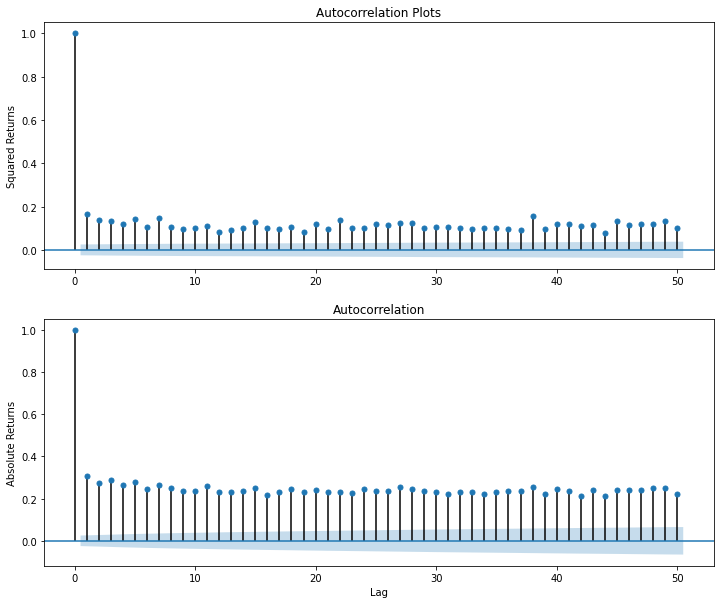### How to plot AMZN stock price 252 days and 21 days moving volatility?

df['moving_std_252'] = df[['log_rtn']].rolling(window=252).std()
df['moving_std_21'] = df[['log_rtn']].rolling(window=21).std()
fig, ax = plt.subplots(31, figsize=(1815),
sharex=True)
df.plot(ax=ax)
ax.set(title='AMZN time series',
ylabel='Stock price (\$)')
df.log_rtn.plot(ax=ax)
ax.set(ylabel='Log returns (%)')
df.moving_std_252.plot(ax=ax, color='r',
label='Moving Volatility 252d')
df.moving_std_21.plot(ax=ax, color='g',
label='Moving Volatility 21d')
ax.set(ylabel='Moving Volatility',
xlabel='Date')
ax.legend()
<matplotlib.legend.Legend at 0x7f2c36643f50>